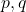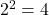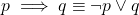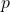# Logical Equivalence

We have seen prepositions, connectives and compound prepositions; all possible combinations of truth values of the individual prepositions in a compound preposition is depicted in a truth-table. However, it is possible that another preposition or compound preposition has the same truth values in the truth table. This is called logical equivalence of two prepositions.

The importance of logical equivalence is in simplifying complex logical expressions. This type of simplification is used in designing digital circuits . Learn digital logic that is the basis for computer system designs.

### Verifying Logical Equivalence using Truth-Table

As we mentioned earlier, the simplest way to verify logical equivalence of two preposition or compound preposition is to create a truth table and compare the output of each logical expression.

The number of variables used in the truth-table for each expression may be different, but we shall only take variables that are common to both the expressions.

Example #1

Suppose you are asked to verify the logical equivalence of following expressions.

p \implies q \equiv \neg p \vee q

The number of unique variables is. Therefore, our truth-table will containrows. The truth-table will contain truth values for all the expressions as given below.

The above table clearly shows that the logically both expressions give the same truth value for all input combinations, that is,is equivalent. Let us see some more examples of logical equivalences.

Example #2

In the second example, we will try to prove the logical equivalence of biconditional connective using truth table.

p \iff q \equiv p \implies q \wedge q \implies p

There are exactly two unique variables in above expressions. Therefore, the truth-table will contain 4 rows.Truth table for logical equivalence p<->q <=> p -> q and q -> p

The truth value for each row is same for both the expressions; therefore, both expressions are equivalent. There are several other logical expressions that we are going to explore in future lessons.